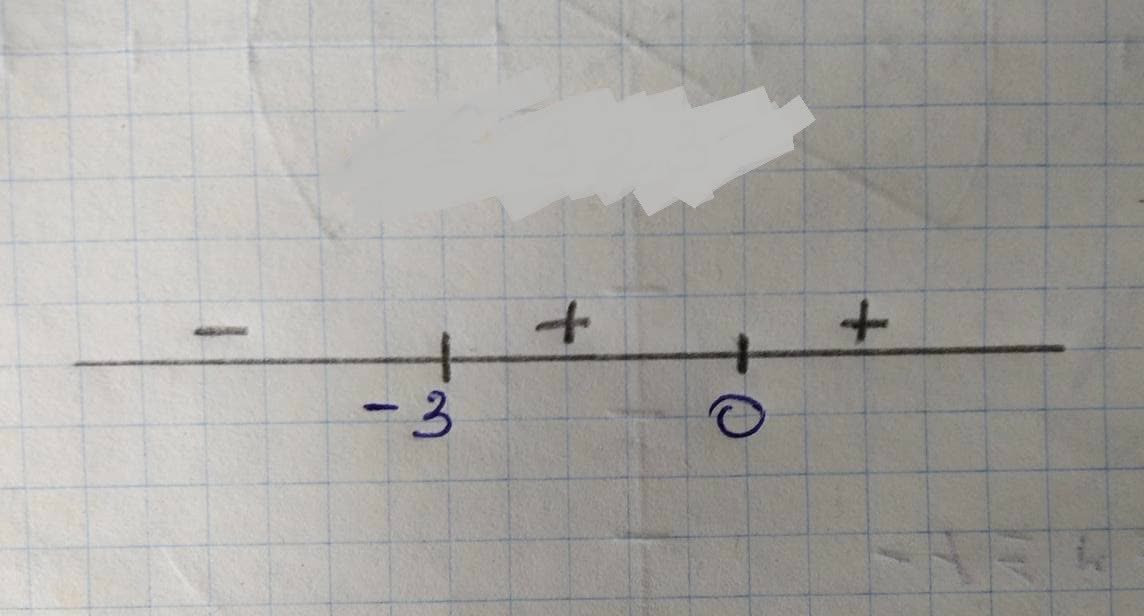# \Let f(x) =x^{5} + 5x^{4} + 6x +3. a. Conduct a sign analysiurca3m403 2021-11-20 Answered
$Letf\left(x\right)={x}^{5}+5{x}^{4}+6x+3.$
a. Conduct a sign analysis of f"". That is, determine the sign off"" on each interval. Make sure to show how you found the boundaries (endpoints) of the intervals and how you computed the sign in each interval.
b. indicate the intervals, if any, on which fis concave up and the intervals, if any, on which fis concave down.
c. Find the x-coordinates of any inflection points of f. If there are more than one, enter them as a comma- separated list. If there are none, enter ""DNE""
You can still ask an expert for help

• Questions are typically answered in as fast as 30 minutes

Solve your problem for the price of one coffee

• Math expert for every subject
• Pay only if we can solve ittnie54
Given:
$F\left(x\right)={x}^{5}+5{x}^{4}+6x+3$
differentioting the above ef wet (x) we heve
${F}^{1}\left(x\right)=5{x}^{4}+20{x}^{3}+6$
again differentiat wet (x),we have
${F}^{11}\left(x\right)=20{x}^{3}+60{x}^{2}$(a.) for beundasies (endpoints) of he interval we apply.
${F}^{11}\left(x\right)=0$
i.e $20{x}^{3}+60{x}^{2}=0$
ov, $20{x}^{2}\left(x+3\right)=0$
The roots of the abore equation are 0,0 8-3
b) for concave up, ${F}^{11}\left(x\right)>0$
So,for $x\succ 3,$ F(x) is concave up.
(c.) Infection points are those points at which
Function changes its concarity that is concave up to concave down ov vice-versa .
In the secHon(a) ,we see that ${F}^{11}\left(x\right)$ cnange its sign once at -3 where concarity changes from concave down to concave up.
So, the point of Inflection is -3.